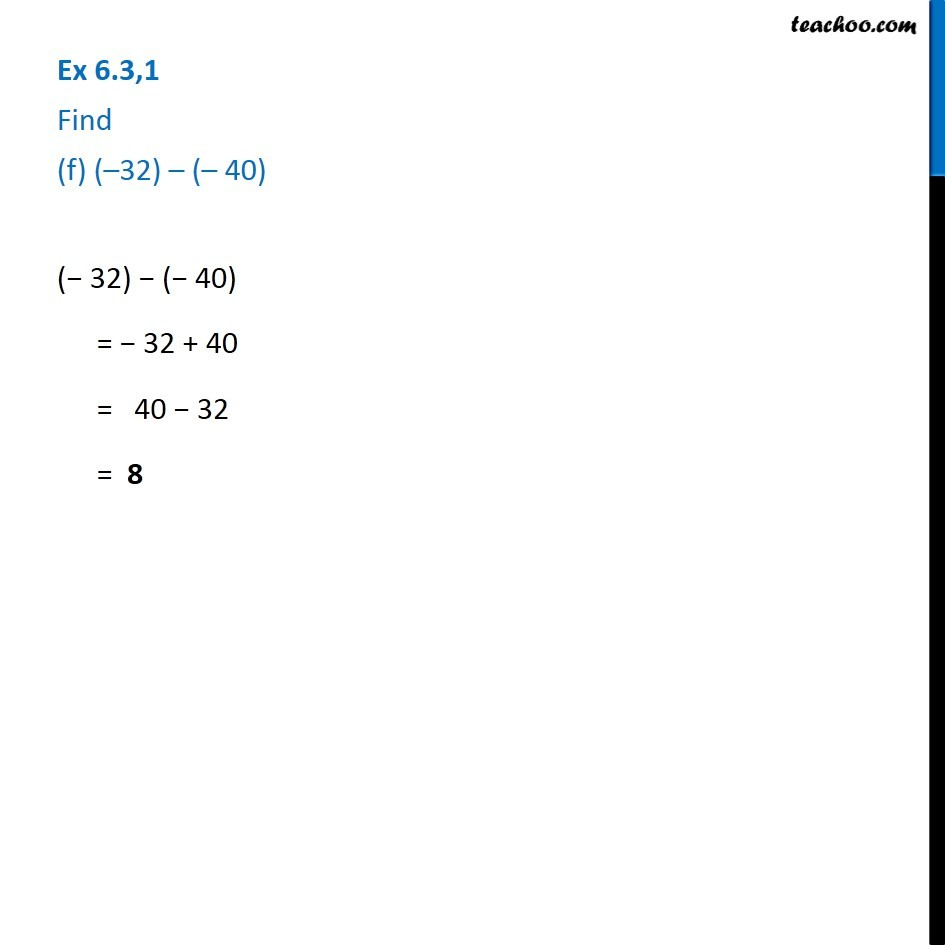1. Chapter 6 Class 6 Integers
2. Serial order wise
3. Ex 6.3

Transcript

Ex 6.3,1 Find (f) (–32) – (– 40) (− 32) − (− 40) = − 32 + 40 = 40 − 32 = 8

Ex 6.3

Chapter 6 Class 6 Integers
Serial order wise